Chapter Three -
Data Descriptions

 Overview:   Measures of Central Tendency   Measures of Variation   Measures of Position   Exploratory Data Analysis

1. Definitions:

Statistic  - A characteristic or measure obtained by using the data values from a sample (This is usually denoted by ROMAN letters)

When I say "ROMAN" letters, I mean "A,B,C,D, etc."

Parameter - A characteristic or measure obtained by using the data values from a population (This is usually denoted by GREEK letters)

When I say "GREEK" letters, I mean (see below):

2. THE GREEK ALPHABET (abbreviated)

 Greek Letter Upper and lower case Spoken as (in English): Special Meaning (in Math): Roman Letter equivalent: A,a "ALPHA" A,a W,w "OMEGA" ("O-MAY-GAH") O,o B,b "BETA" ("BAY-TAH") B,b G,g "GAMMA" G,g D,d "DELTA" Expresses a change in a quantity D,d E,e "EPSILON" E,e P,p "PI" Either "Multipication", or  the special number in geometry "3.1415~" P,p S,s "SIGMA" Addition S,s Q,q "THETA" ("THAY-TAH") Geometric Angle Th (as in "the")

These are just a few. There are
These symbols may seem strange, but the SIGMA will be VERY important, as you will see soon.

3. HOW DO YOU FIND THE MIDDLE GROUND?

Measures of Central Tendency

The basic idea here is that, in a given data set, we want to know where the "middle of the pack" is located. There are four different ways to do this. They are:

• The Mean
• The Median
• The Mode
• The Midrange
Think of it this way: You know the expression "behind the power curve". How do we know if we are ahead , behind, or right on the "power curve"?  In order to know this, we need to have a good , numerical measurement of where the majority of our data points are located. It might be that the high score is a 100% on a test, and the lowest is a 60%.  But what if a majority of the students got at least a 70%, and no better than 75%? The mean, median, the mode, and the midrange help us figure out the boundaries of the "power curve".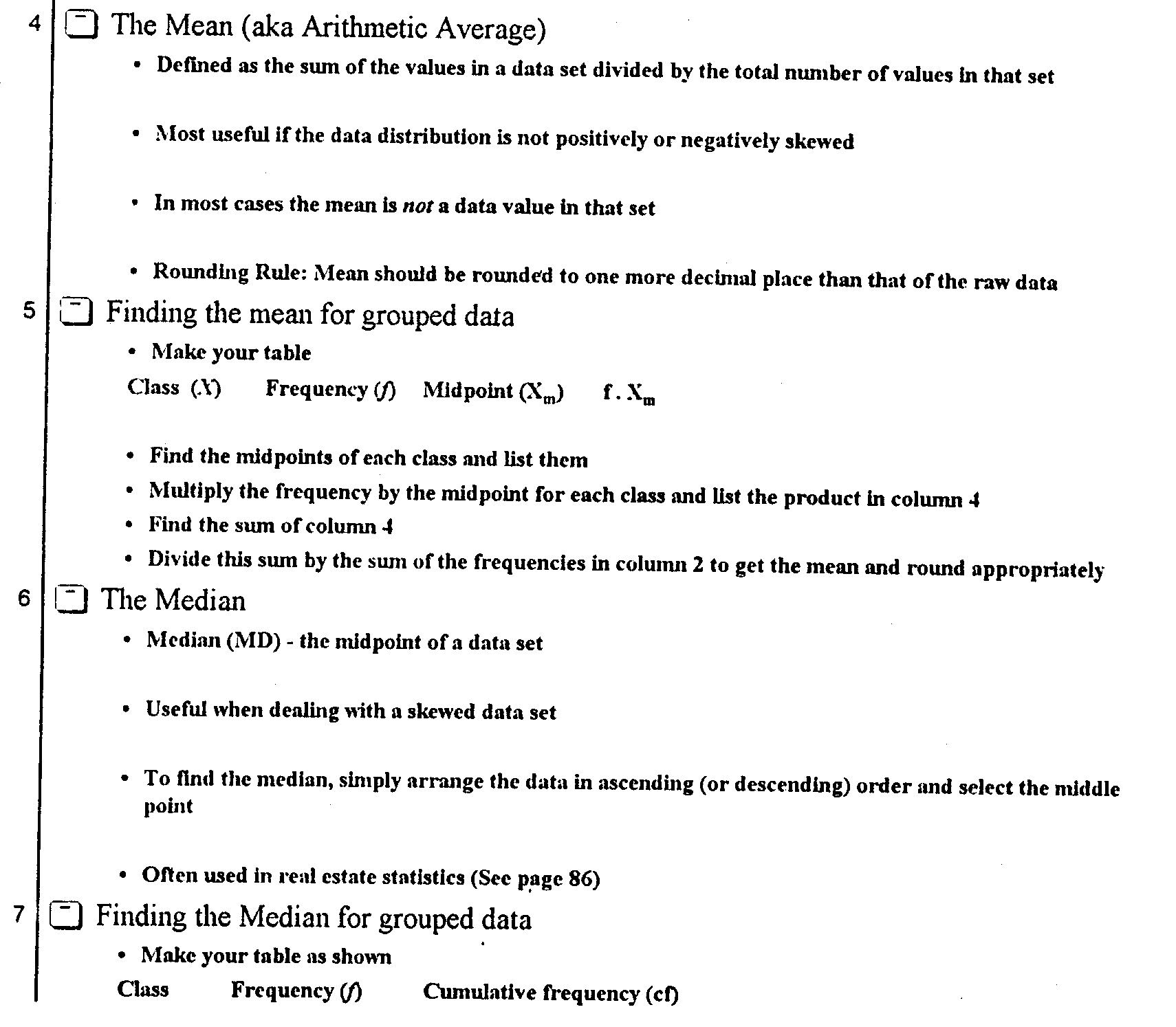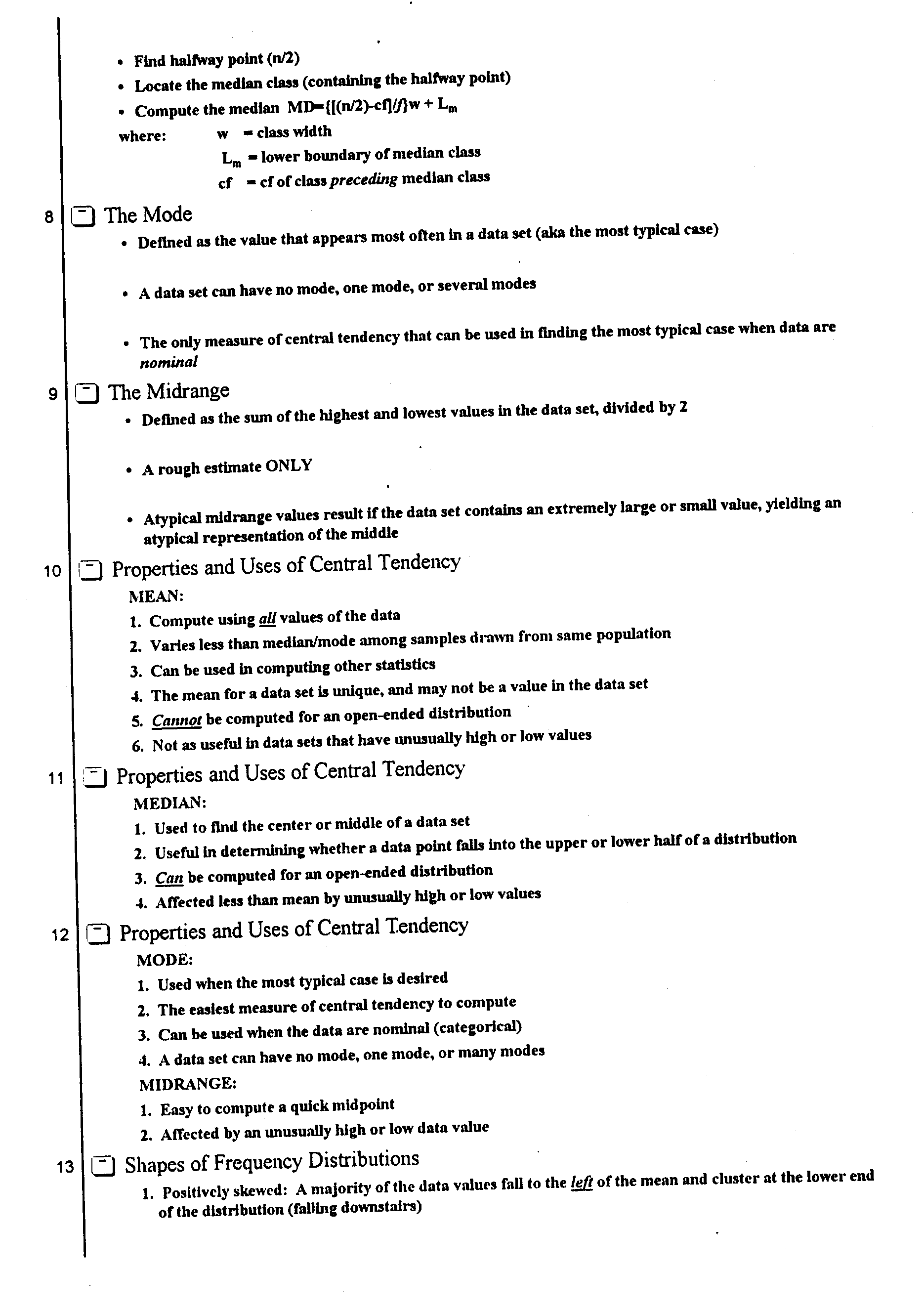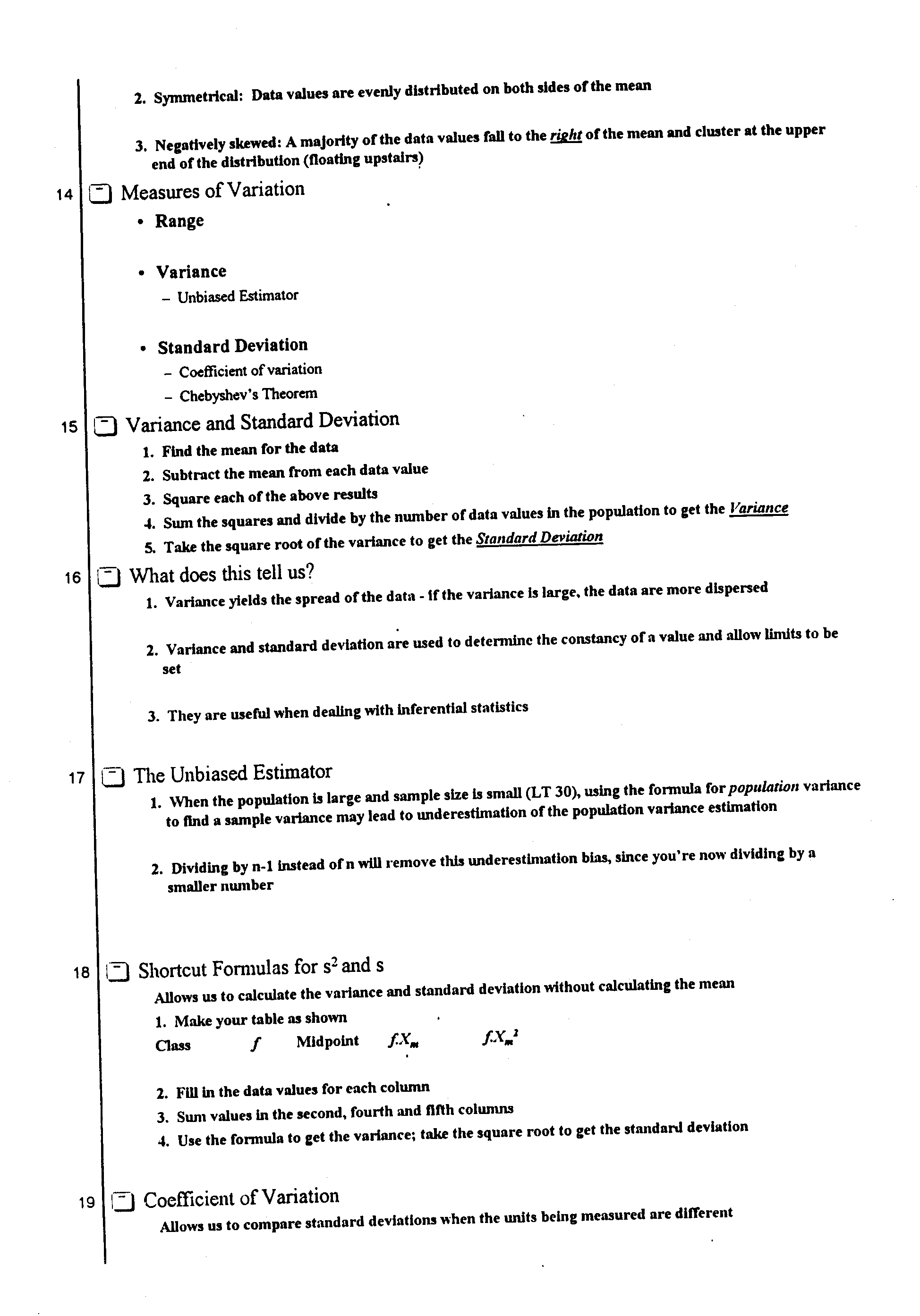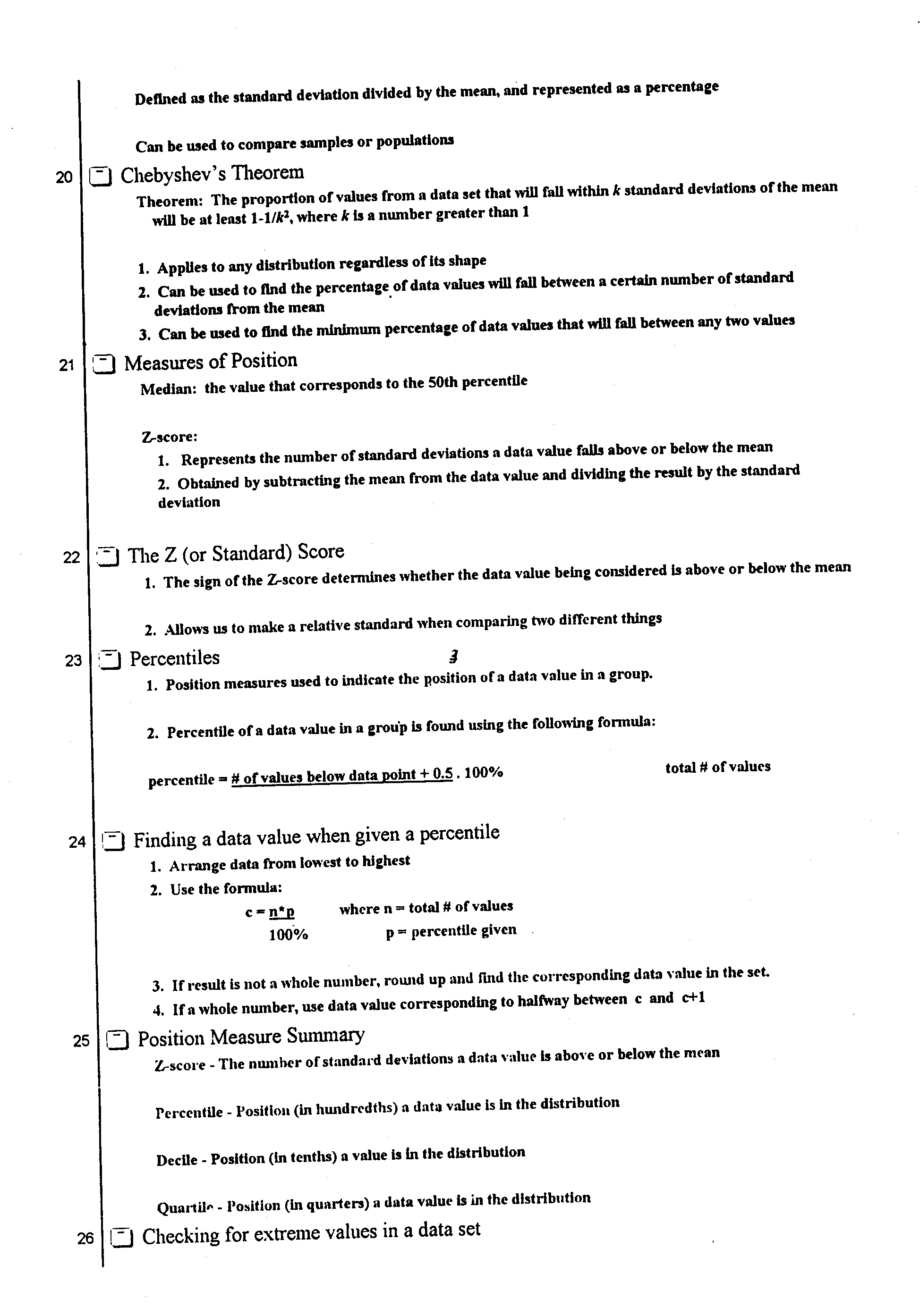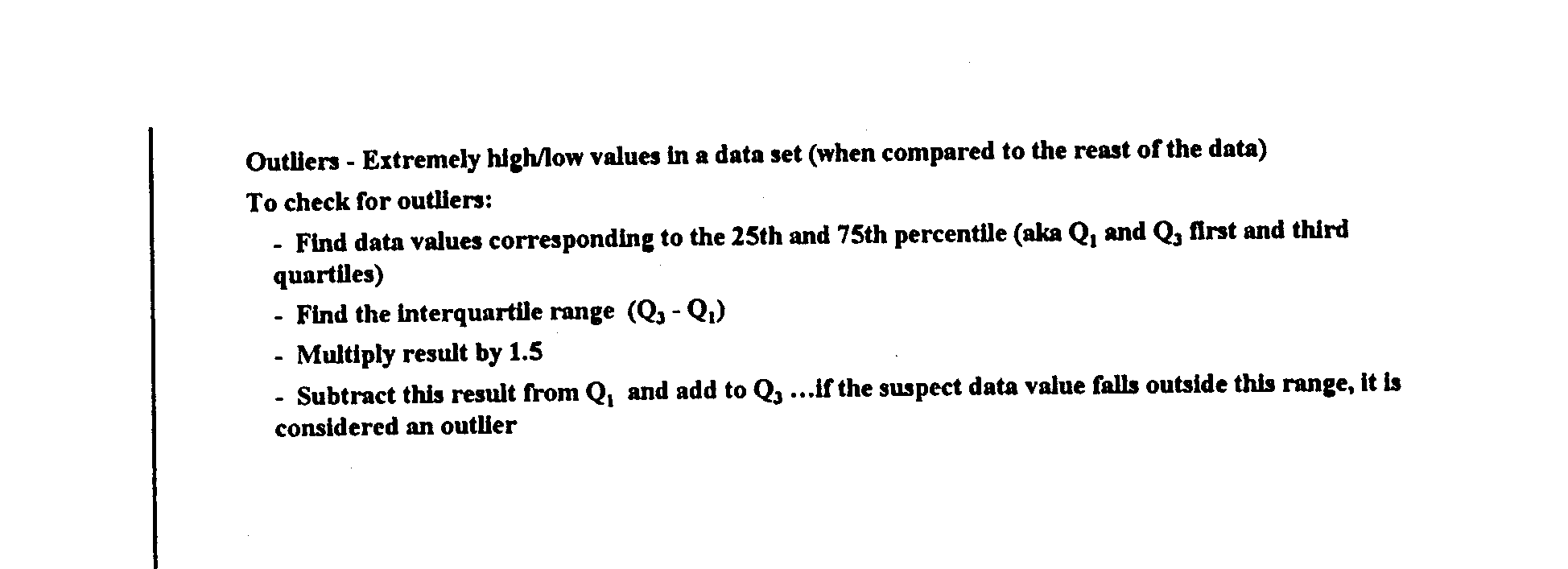HOMEWORK:   Read Chapter 3 in the textbook